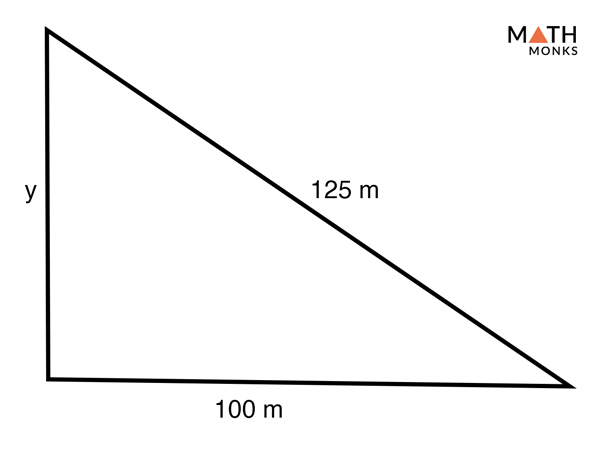# 3-4-5 Triangle

As we know, special right triangles can have different dimensions, but among them, the most common is the 3-4-5 right triangle. Here, we will learn about 3-4-5 right triangles and how to solve problems involving them.

## What is a 3-4-5 Triangle

A 3-4-5 triangle is a special right triangle whose side lengths are in the ratio of 3: 4: 5. It is thus a right triangle with sides in the ratio of integer lengths (whole numbers) called Pythagorean triples. Since all its side lengths are different from the other; it is also called a scalene-right triangle.

Being a right triangle, the Pythagoras formula a2 + b2 = c2, where a = side 1, b = side 2, and c = hypotenuse is also applicable in a 3-4-5 triangle.

In a 3-4-5 triangle,

a2 + b2 = c2, here a = 3 units, b = 4 units

⇒ 32 + 42 = c2

⇒ 25 = c2

⇒ c = 5 units

Hence, it works.

Other Pythagorean Triples

3-4-5 triangle does not mean that the ratios are always exactly 3: 4: 5. But, it can be any factor of numbers, keeping the basic ratio of the three sides the same. Few other examples of 3-4-5 triangles are:

• 6-8-10
• 9-12-15
• 12-16-20
• 15-20-25

## Properties

1. All three sides are unequal, having a ratio of 3: 4: 5 (3x: 4x: 5x for Side 1: side 2: hypotenuse).
2. All three internal angles are unequal measuring 36.87°, 53.13°, and 90°

## How to Solve a 3-4-5 Triangle

Solving a 3-4-5 right triangle involves finding the missing side lengths of the triangle. The ratio of 3: 4: 5 allows us to calculate the unknown lengths without using the Pythagorean Theorem or trigonometric functions.

Find the length of the unknown side of a right triangle in which hypotenuse measures 35 cm and one of the other side measures 28 cm.

Solution:

We first have to prove whether the given triangle is a 3-4-5 triangle, for which the test ratio should be 3:4:5.
?: 28: 35 = ?: 4(7): 5(7)
Here, factor x = 7
Thus, the given triangle is a 3-4-5 right triangle
Hence, the length of the unknown side is:
3x = 3 x 7 = 21 cmFind the value of y in the given triangle. Assume that the triangle is a 3-4-5 right triangle.

Solution:

We first have to prove whether the given triangle is a 3-4-5 triangle, for which the test ratio should be 3:4:5
?: 100: 125 = ?: 4(25): 5(25)
Here, factor x = 25
Thus, the given triangle is a 3-4-5 right triangle
Hence, the length of the unknown side (y) is:
y = 3x = 3 x 25 = 75 cm

Calculate the length of the diagonal of a right triangle with side lengths of 9mm and 12mm.

Solution:

We first have to prove whether the given triangle is a 3-4-5 triangle, for which the test ratio should be 3:4:5
9: 12: ? = 3(3): 4(3): ?
Here, factor x = 3
Thus, the given triangle is a 3-4-5 right triangle,
Substituting the value of x, we get
Diagonal = hypotenuse = 5x = 5 x 3 =15mm
Hence, the length of the diagonal is 15mm.

The longest edge and bottom edge of triangular-shaped sail of a boat is 20m and 16m respectively. Find the height of the sail?

Solution:

Since, we first need to check whether the triangular sail makes a 3-4-5 right triangle with the bottom, we will find its test ratio.
⇒ ?: 16: 20 = ? : 4(4): 5(4)
Here, factor x = 4
Thus, the given triangle is a 3-4-5 right triangle
Substituting the value of x, we get Height = 3x = 3 x 4 = 12m
Hence, the height of the sail is 12m.